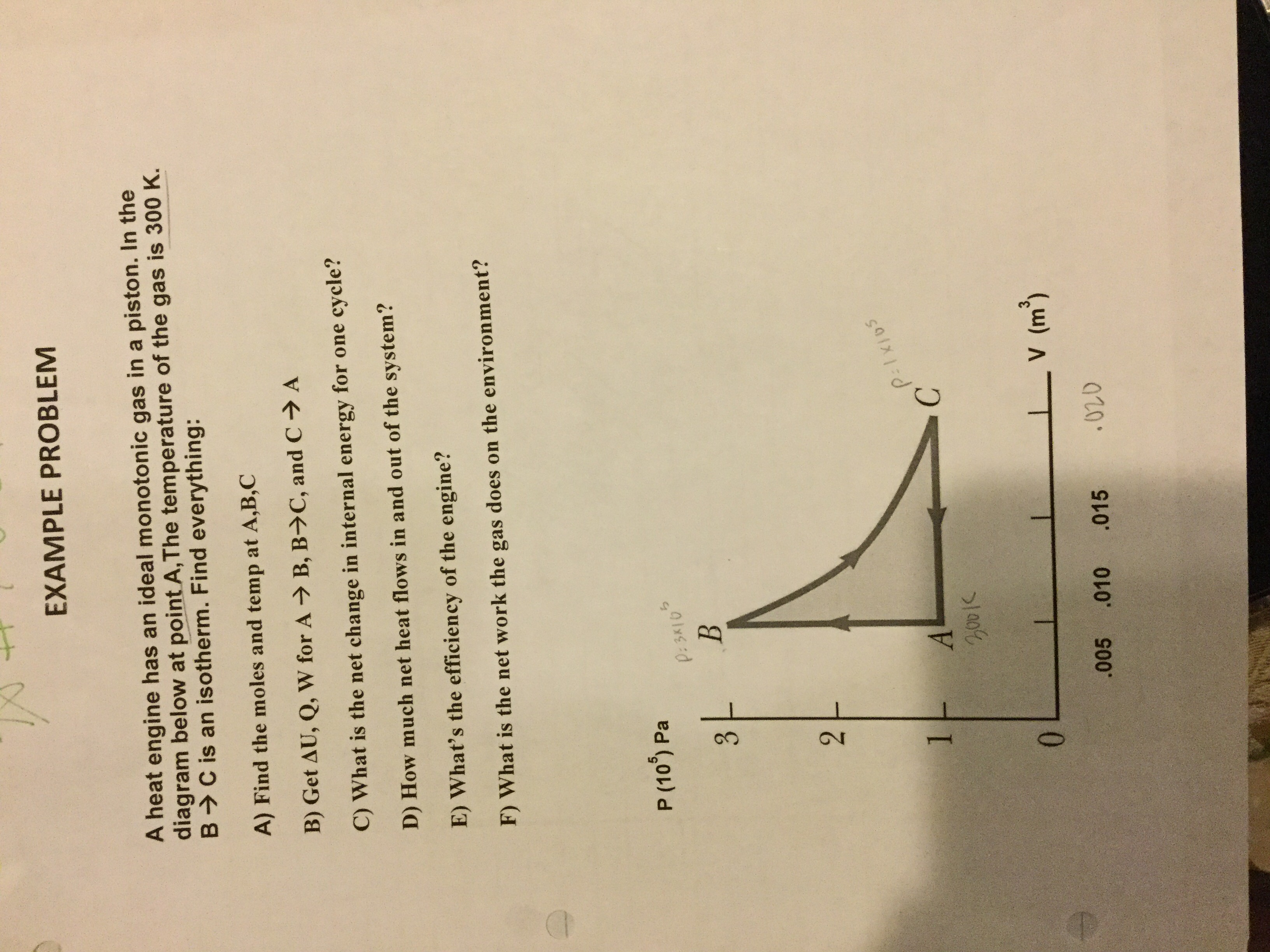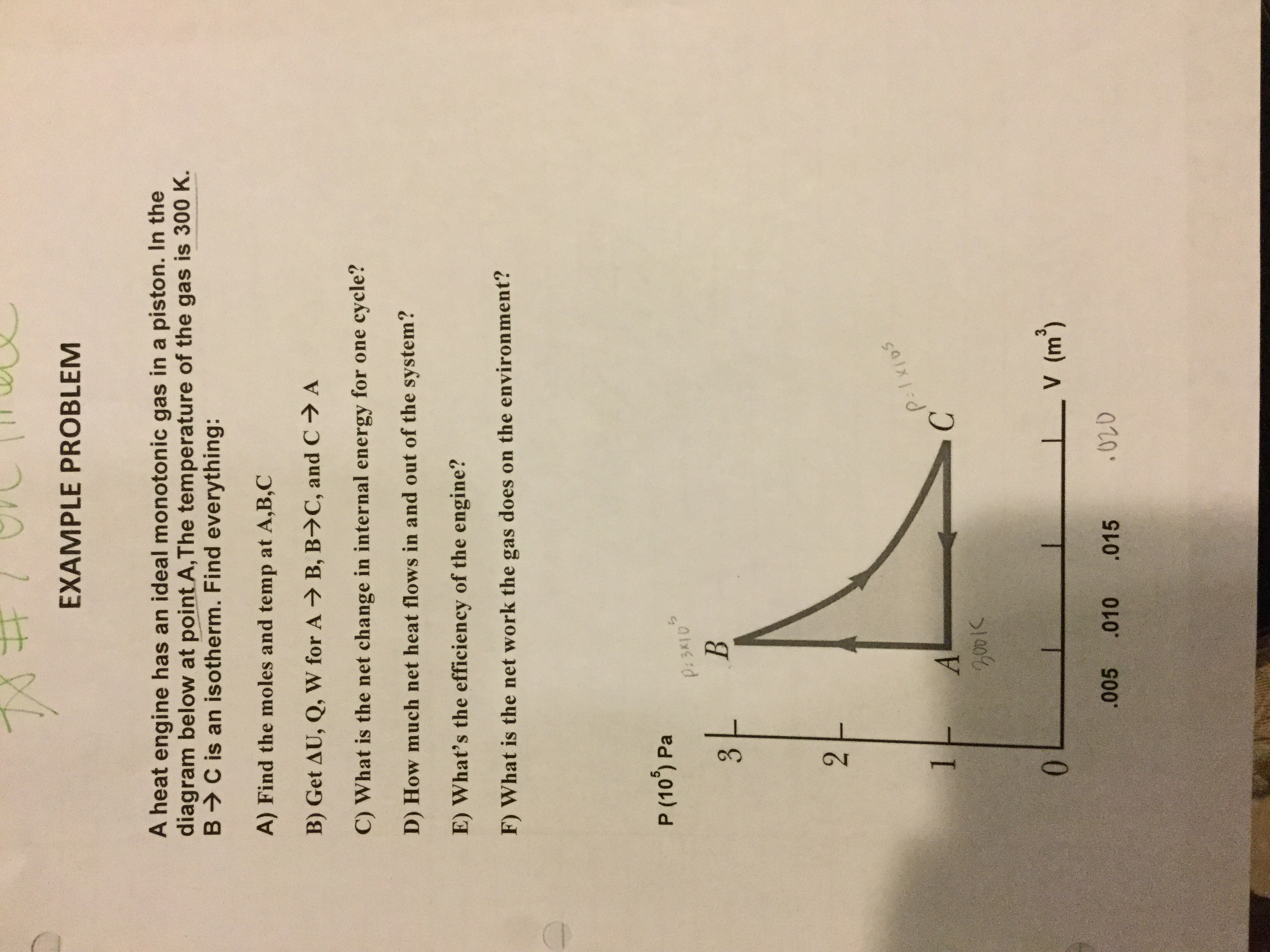# 3.2,EXAMPLE PROBLEMA heat engine has an ideal monotonic gas in a piston. In thediagram below at point A,The temperature of the gas is 300 K.B> C is an isotherm. Find everything:A) Find the moles and temp at A,B,CB) Get AU, Q, W for AB, B>C, and C→ AC) What is the net change in internal energy for one cycle?D) How much net heat flows in and out of the system?E) What's the efficiency of the engine?F) What is the net work the gas does on the environment?P (10°) PaP:3K10P:1x10s1.C.I V (m).005.010.015 3.EXAMPLE PROBLEMA heat engine has an ideal monotonic gas in a piston. In thediagram below at point A,The temperature of the gas is 300 K.B>C is an isotherm. Find everything:A) Find the moles and temp at A,B,CB) Get AU, Q, W for AB, B>C, and C→ AC) What is the net change in internal energy for one cycle?D) How much net heat flows in and out of the system?E) What's the efficiency of the engine?F) What is the net work the gas does on the environment?P (10) PaP:3K102.P:1xios1C.0..005LV (m³).010.015

Question
4 views

Can you help me?help_outlineImage Transcriptionclose3. 2, EXAMPLE PROBLEM A heat engine has an ideal monotonic gas in a piston. In the diagram below at point A,The temperature of the gas is 300 K. B> C is an isotherm. Find everything: A) Find the moles and temp at A,B,C B) Get AU, Q, W for A B, B>C, and C→ A C) What is the net change in internal energy for one cycle? D) How much net heat flows in and out of the system? E) What's the efficiency of the engine? F) What is the net work the gas does on the environment? P (10°) Pa P:3K10 P:1x10s 1. C. I V (m) .005 .010 .015 fullscreenhelp_outlineImage Transcriptionclose3. EXAMPLE PROBLEM A heat engine has an ideal monotonic gas in a piston. In the diagram below at point A,The temperature of the gas is 300 K. B>C is an isotherm. Find everything: A) Find the moles and temp at A,B,C B) Get AU, Q, W for A B, B>C, and C→ A C) What is the net change in internal energy for one cycle? D) How much net heat flows in and out of the system? E) What's the efficiency of the engine? F) What is the net work the gas does on the environment? P (10) Pa P:3K10 2. P:1xios 1 C. 0. .005 LV (m³) .010 .015 fullscreen
check_circle

Step 1

a)

at A temperature is 300 K,

at A, the number of moles is

Step 2

substitute the known values in the above equation,

Step 3

at B ,

for isobar...

### Want to see the full answer?

See Solution

#### Want to see this answer and more?

Solutions are written by subject experts who are available 24/7. Questions are typically answered within 1 hour.*

See Solution
*Response times may vary by subject and question.
Tagged in

### Science